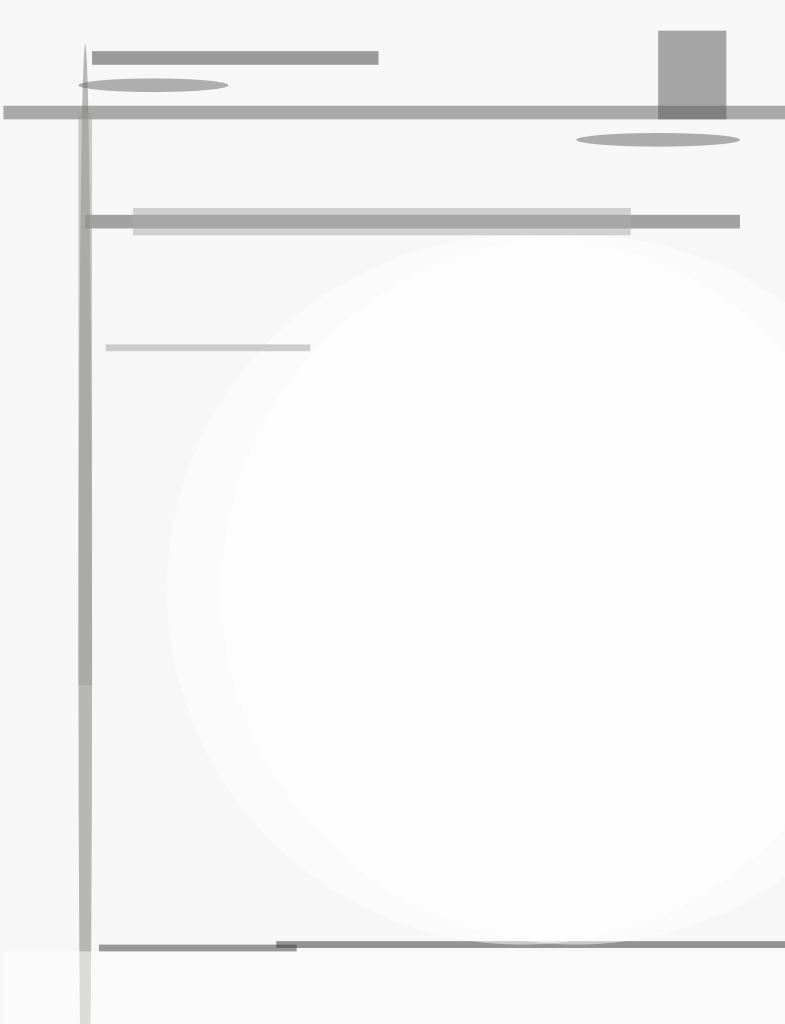A prototype Digital Waveform Generator (DWG) in the ultra-high-frequency (UHF) range uses Delta-Sigma (Δ−Σ) modulation techniques, which permit arbitrary and accurate waveform generation. The DWG meets the demanding and diverse waveform requirements of future radar applications, including linear-FM (LFM) and continuous wave (CW) signals. This DWG also allows for the generation of waveforms at other frequencies by up-conversion or down-conversion.

The Δ-Σ filter algorithm or Δ−Σ modulator is used to generate a single-bit waveform from the digital input data with a CW center frequency between 406 and 450 MHz, both with and without a 4 MHz bandwidth LFM, with a nominal pulsewidth of 10 μs and an available filter bandwidth of 50 MHz.

A simple, first-order modulator is shown in the figure. The output of the comparator, V(z), is also the output of the loop, which is full-scale ±1 V. At the summation, either +1 V or -1 V is added to the input voltage. The result is the input to the Δ−Σ digital filter. The digital filter can implement the filter equation using the two fundamental arithmetic operations, multiplication and addition (or accumulation). If the output of the digital filter, Y(z), is greater than 0 V, V(z) becomes +1 V; if it is less than 0 V, V(z) becomes -1 V. A no-change condition causes the modulated signal to remain at the same ±1 V state of the previous sample. Each operation occurs once during each clock cycle. The output of the loop, either +1 V or -1 V, will be saved in a single-bit data file as either a 1 or 0, respectively. Then the digital single-bit data series is converted to an analog signal by using a high-speed single-bit DAC. In general, first- and second-order modulators are stable, but stability must be considered in higher-order units.

Both transposed and hybrid Δ−Σ approaches were considered. The approaches included a comparison of transposed filters of various orders. The transposed filter quantization error in higher-order (e.g., M = 8) filters was considered and hybrid filters of various orders were compared. The transposed filter has a short critical path of two full additions and one multiply. It thus has the advantage of a short critical path and an efficient finite-impulse response (FIR) implementation. It is, however, extremely sensitive to coefficient quantization accuracy. Transposed filters were analyzed for three orders (M = 2, 4, 8). In particular, the sensitivity of quantization error vs signal-to-noise ratio (SNR) was investigated.

The Δ−Σ hybrid filter has the same critical path as the transposed filter. With the use of higher-order filters such as with M = 16, coefficient quantization reduces the SNR to an unacceptable level.

This work was done by Dr. Lawrence M. Leibowitz of SFA, Inc., and Sukomal Talapatra and Jimmy O. Alatishe of the Surveillance Technology Branch Radar Division of the Naval Research Laboratory.

NRL-0030

##### This Brief includes a Technical Support Package (TSP).